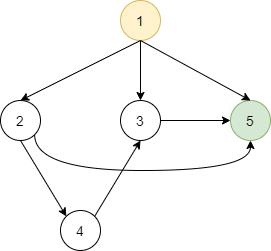Home Practice Programming Count all possible paths between two vertices of a directed graph

# Count all possible paths between two vertices of a directed graph

Given a directed graph G, and two vertices: start s & end e, count all possible way of reaching the vertex e from vertex s. Note, these paths don’t contain cycle as the existence of the cycle would lead to an infinite number of paths.The total possible ways of reaching vertex 5 from vertex 1 in the above-directed graph are 4.

Consider the above graph. Here 1 is the start vertex and 5 is the ending vertex. The total number of the possible paths between two vertices 1 & 5 is 4.

```Explanation
Total number of ways of reaching vertex 5 from vertex 1 -
1)  1 -> 5
2)  1 -> 2 -> 5
3)  1 -> 3 -> 5
4)  1 -> 2 -> 4 -> 3 -> 5```

#### Approach

To solve the above problem we will DFS with a slight modification because DFS will only be able to find if there exists a path between two vertices or not. It won’t be able to give us the count of the total number of paths possible.

The Depth First Search(DFS) is a recursive algorithm used to traverse a Graph. The algorithm starts with an arbitrary node(in case of a graph) and traverses all the nodes of the graph in a depth-first manner. It uses a stack to store the intermediate visited nodes to prevents the algorithm from falling into an infinite loop.

The modification we will do to the existing DFS is to mark the current vertex unvisited after all of its children are visited.

#### Algorithm

1. Create a recursive function dfs() that takes the index of the source node and the destination node of a directed graph and keep a global variable to store the count.
2. If the current node is the destination node, then increase the value of the count variable by 1.
3. Else, if the current node is not visited, mark it visited, call the recursive function dfs() on all of its child nodes, and once the recursive calls complete, mark the current node as unvisited again.
4. Return the value of the variable count i.e. total possible paths between two vertices
```dfs(graph, start, destination, visited):
if(start == destination):
count = count+1
return

if(visited[start] == true):
return

visited[s] = true
for(all the childs of current vetex):
if(visited[child] == false):
dfs(graph, child, destination, visited);

visited[start] = false
return
}
```

#### Implementation of the above algorithm

```/* C++ program to count all the possible paths
from a source to a destination */

#include <bits/stdc++.h>
using namespace std;

/* Modified dfs unction to count the possible
paths between 2 vertices */
void dfs(list<int> graph[], int s, int d, int *cnt, bool *visited)
{
/*If we have reached the destination vetex
then increase the path count by 1 */
if(s == d){
*cnt = *cnt+1;
return ;
}

if(visited[s] == true)
return;

/*Mark the current vertex as visited*/
visited[s] = true;
list<int>::iterator it;

/* Call to the modified dfs function on
all the child nodes of the current vetex */
for( it = graph[s].begin() ; it != graph[s].end() ; it++)
{
if(visited[*it] == false)
dfs(graph, *it, d, cnt, visited);
}

/*Mark the current vertex as unvisited*/
visited[s] = false;

return;
}

// Function to count the possible paths between 2 vertices
int countPaths(list<int> graph[], int V, int s, int d)
{
//Array to store visited vertices
bool visited[V+1] = {false};

//variable to count total number
//of possible path b/w two vertices
int count = 0;

/*call to the modified DFS function with
count variable passed by reference */
dfs(graph, s, d, &count, visited);

//return the possible paths between 2 vertices
return count;
}

//Main Driver Code
int main() {

int n = 5;
int s = 1, d = 5;
list <int> *adj = new list <int>[n + 1];

cout << countPaths(adj, n, s, d) << endl;

return 0;
}

```
```Output
4```

#### Time Complexity

The run time complexity of the above algorithm is O(V+E), which is equivalent to that of DFS, where E is total no. of edges and V is total no. of vertices.

The space complexity of the above algorithm is O(V), because of the extra visited vetex storage.